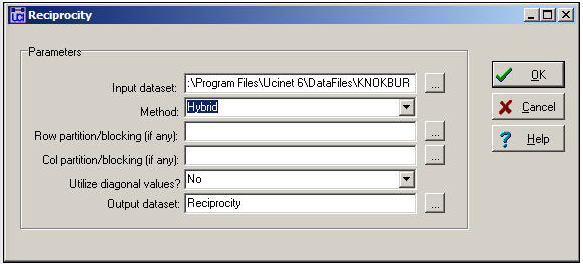$$\newcommand{\id}{\mathrm{id}}$$ $$\newcommand{\Span}{\mathrm{span}}$$ $$\newcommand{\kernel}{\mathrm{null}\,}$$ $$\newcommand{\range}{\mathrm{range}\,}$$ $$\newcommand{\RealPart}{\mathrm{Re}}$$ $$\newcommand{\ImaginaryPart}{\mathrm{Im}}$$ $$\newcommand{\Argument}{\mathrm{Arg}}$$ $$\newcommand{\norm}{\| #1 \|}$$ $$\newcommand{\inner}{\langle #1, #2 \rangle}$$ $$\newcommand{\Span}{\mathrm{span}}$$

# 8.3: Reciprocity

$$\newcommand{\vecs}{\overset { \rightharpoonup} {\mathbf{#1}} }$$ $$\newcommand{\vecd}{\overset{-\!-\!\rightharpoonup}{\vphantom{a}\smash {#1}}}$$$$\newcommand{\id}{\mathrm{id}}$$ $$\newcommand{\Span}{\mathrm{span}}$$ $$\newcommand{\kernel}{\mathrm{null}\,}$$ $$\newcommand{\range}{\mathrm{range}\,}$$ $$\newcommand{\RealPart}{\mathrm{Re}}$$ $$\newcommand{\ImaginaryPart}{\mathrm{Im}}$$ $$\newcommand{\Argument}{\mathrm{Arg}}$$ $$\newcommand{\norm}{\| #1 \|}$$ $$\newcommand{\inner}{\langle #1, #2 \rangle}$$ $$\newcommand{\Span}{\mathrm{span}}$$ $$\newcommand{\id}{\mathrm{id}}$$ $$\newcommand{\Span}{\mathrm{span}}$$ $$\newcommand{\kernel}{\mathrm{null}\,}$$ $$\newcommand{\range}{\mathrm{range}\,}$$ $$\newcommand{\RealPart}{\mathrm{Re}}$$ $$\newcommand{\ImaginaryPart}{\mathrm{Im}}$$ $$\newcommand{\Argument}{\mathrm{Arg}}$$ $$\newcommand{\norm}{\| #1 \|}$$ $$\newcommand{\inner}{\langle #1, #2 \rangle}$$ $$\newcommand{\Span}{\mathrm{span}}$$

With symmetric dyadic data, two actors are either connected, or they are not. Density tells us pretty much all there is to know.

With directed data, there are four possible dyadic relationships: A and B are not connected, A sends to B, B sends to A, or A and B send to each other. A common interest in looking at directed dyadic relationships is the extent to which ties are reciprocated. Some theorists fell that there is an equilibrium tendency toward dyadic relationships to be either null or reciprocated, and that asymmetric ties may be unstable. A network that has a predominance of null or reciprocated ties over asymmetric connections may be a more "equal" or "stable" network than one with a predominance of asymmetric connections (which might be more of a hierarchy).

There are (at least) two different approaches to indexing the degree of reciprocity in a population. Consider the very simple network shown in Figure 8.3. Actors A and B have reciprocated ties, actors B and C have a non-reciprocated tie, and actors A and C have no tie.Figure 8.3: Definitions of reciprocity

What is the prevalence of reciprocity in this network? One approach is to focus on the dyads, and ask what proportion of pairs have a reciprocated tie between them? This would yield one such tie for three possible pairs (AB, AC, BC), or a reciprocity rate of 0.333. More commonly, analysts are concerned with the ratio of the number of pairs with a reciprocated tie relative to the number of pairs with any tie. In large populations, usually most actors have no direct ties to most other actors, and it may be more sensible to focus on the degree of reciprocity among pairs that have any ties. In our simple example, this would yield one reciprocated pair divided by two tied pairs, or a reciprocity rate of 0.500. The method just described is called the dyad method in Network>Cohesion>Reciprocity.

Rather than focusing on actors, we could focus on relations. We could ask, what percentage of all possible ties (or "arcs" of the direct graph) are parts of reciprocated structures? Here, two such ties (A to B and B to A) are a reciprocated structure among the six possible ties (AB, BA, AC, CA, BC, CB) or a reciprocity of 0.333. Analysts usually focus, instead, on the number of ties that are involved in reciprocal relations relative to the total number of actual ties (not possible ties). Here, this definition would give us 2/3 or 0.667. This approach is called the arc method in Network>Cohesion>Reciprocity. Here's a typical dialog for using this tool.Figure 8.4: Dialog for Network>Cohesion>Reciprocity

We've specified the "hybrid" method (the default) which is the same as the dyad approach. Note that it is possible to block or partition the data by some pre-defined attribute (like in the density example previously) to examine the degree of reciprocity within and between sub-populations. Figure 8.5 shows the results for the Knoke information network.Figure 8.5: Reciprocity in the Knoke information network

We see that, of all pairs of actors that have any connection, $$53\%$$ of the pairs have a reciprocated connection. This is neither "high" nor "low" in itself, but does seem to suggest a considerable degree of institutionalized horizontal connection within this organizational population.

The alternative method of "arc" reciprocity (not shown here) yields a result of 0.6939. That is, of all the relations in the graph, $$69\%$$ are parts of reciprocated ties.